Chapter 10

# Chapter 10

Chapter 10:-

## Income Determination

Plus Two Economics Chapter 10 Income Determination## Introduction

The basic objective of macro-economics is to develop theoretical tools or models. The models attempt to provide theoretical explanation to questions. such as what causes periods of slow growth or recession in the economy or increase in price level, or a rise in unemployment. In this chapter we discuss determination of aggregate output, price level, employment, etc., in the economy.

## Income and Employment

Adam smith, David Ricardo, Jean Baptist Say etc. were the propagators of classical economic thought. The classicalist believed that the economy should be free from controls and the intervention of the government. The role of government in such economies should be very minimum. Such a free economy should always be in equilibrium and attains full employment of resources. They treat full employment as a normal situation and any deviation from this level is something abnormal, which automatically tends towards full employment.

The classicalists framed these ideas based on the economic thoughts of J.B Say. As we have stated earlier, Say’s law of market advocates the theory of ‘supply always creates its own demand’.

## Income and Employment: Keynesian Approach

It was J. M Keynes, who proved that the concept of Say’s law of market was practically wrong, The great depression of 1930’s and the subsequent economic events confirmed his arguments. Keynes’ ideas were published in his epoch-making book entitled General Theory of Employment, Interest and Money published in 1936. It revolutionized the existing economic thinking. He totally denied the classical concept of full employment. He argued that an economy can reach the equilibrium position even without full employment, which is known as the under employment situation.

Effective demand is the basis of Keynesian principles. He argued that the level of income and employment in an economy depends on effective demand. Lack of effective demand leads to unemployment. Effective demand is reached at a point where aggregate demand equals aggregate Supply.

## Equation and Graph

Equations and graphs are inevitable in economics. We can easily understand the relation between economic variables through equations and graphs. Here we shall discuss the most frequently used equation in economics – the Linear equation.

y =a + bx is a linear equation, because its graph will be a straight line as shown in below Diagram.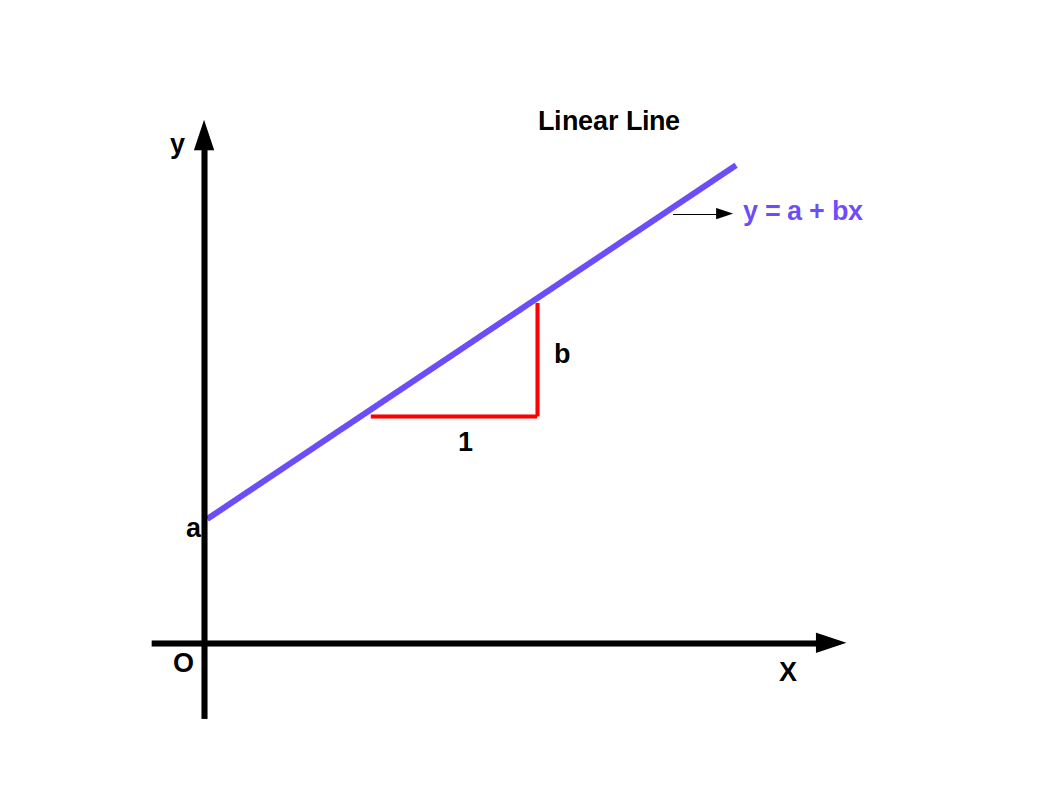In this equation x and y are two Variables. The variable on the left side of the equation is called dependent variable and the variable on the right is called independent variable, a is the vertical intercept and b is the slope. When value of x is 0, value of y is a and when. value of x changes by 1 unit the change in the value of y is b.

## Movement Along a Curve versus Shift of a Curve

Change in the variables of a graph/equation is called movement along a curve. That is, when there is a change of one unit in x, there will be b unit change in y. This is called movement along the curve.

The change in the position of the graph due to change in the para- meters is called parametric shift. Parameters a and b regulate the , position of the curve. When the parameters a and b change, the curve shifts upwards or downwards. This is known as parametric shift. If the value of ‘a’ increases the curve shifts parallel upwards, and if its value decreases the curve shifts parallel downwards. If the value of ‘b’ increases the curve swings upwards, if the value of ‘b’ decreases, the curve swings downwards.

Consider the equation y = 2 + 0.5x, where a and b have fixed values. If we give different values for x, the change in the value of y is given in the following table:

Table 10.1
x y = 2 + 0.5x Method of finding y
0 2 y = 2+0.5×0 = 2+0 = 2
1 2.5 y = 2+0.5×1 = 2+.5 = 2.5
2 3 y = 2+0.5×2 = 2+1 = 3
3 3.5 y = 2+0.5×3 = 2+1.5 = 3.5

When x = 0, the value of y is vertical intercept. This is 2. The slope of the graph is 0.5. Equation y = 2 + .5x is given in below Graph.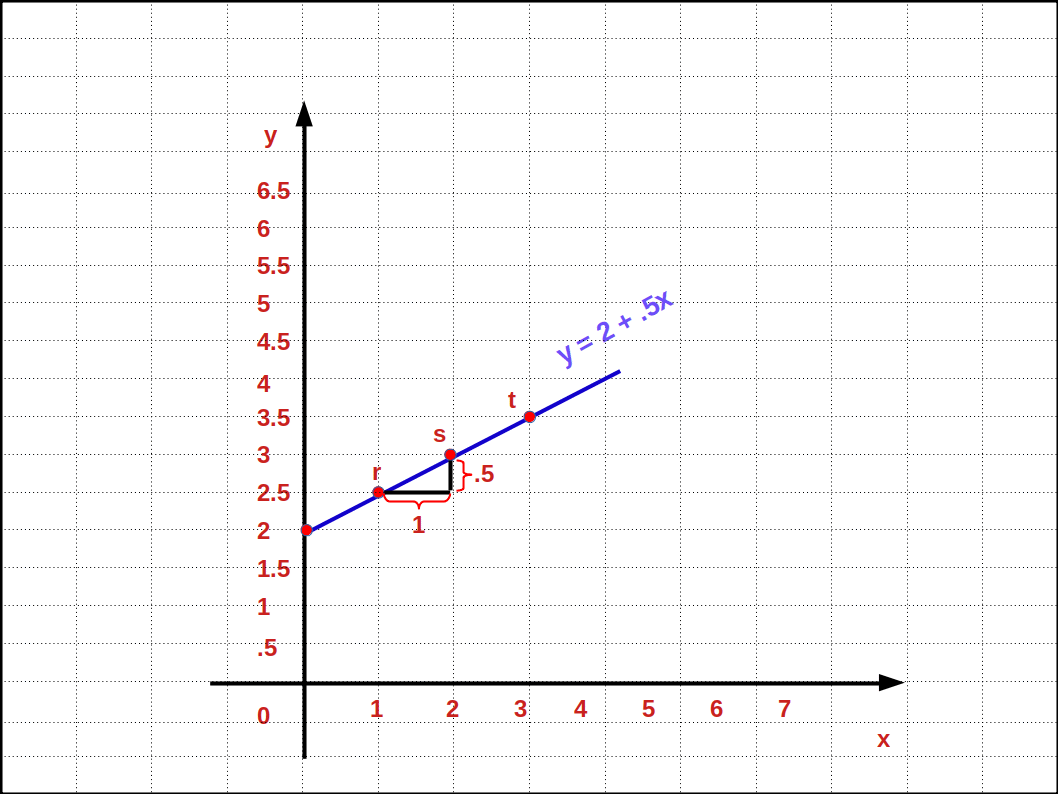When the value of x increases as 1, 2 and 3, the curve moves through the pointy, s, t on the curve. Similarly, when the value of x decreases as 3, 2, 1 it moves downwards through the points t, s, r. This change is called movement along the curve.

Suppose keeping the value of b as .5, the value of intercept a inereases from 2 to 3. Then two straight lines are formed equal to these values. As a result the straight line with intercept 3 shifts up parallel to the straight line with intercept 2 as shown in the below Graph.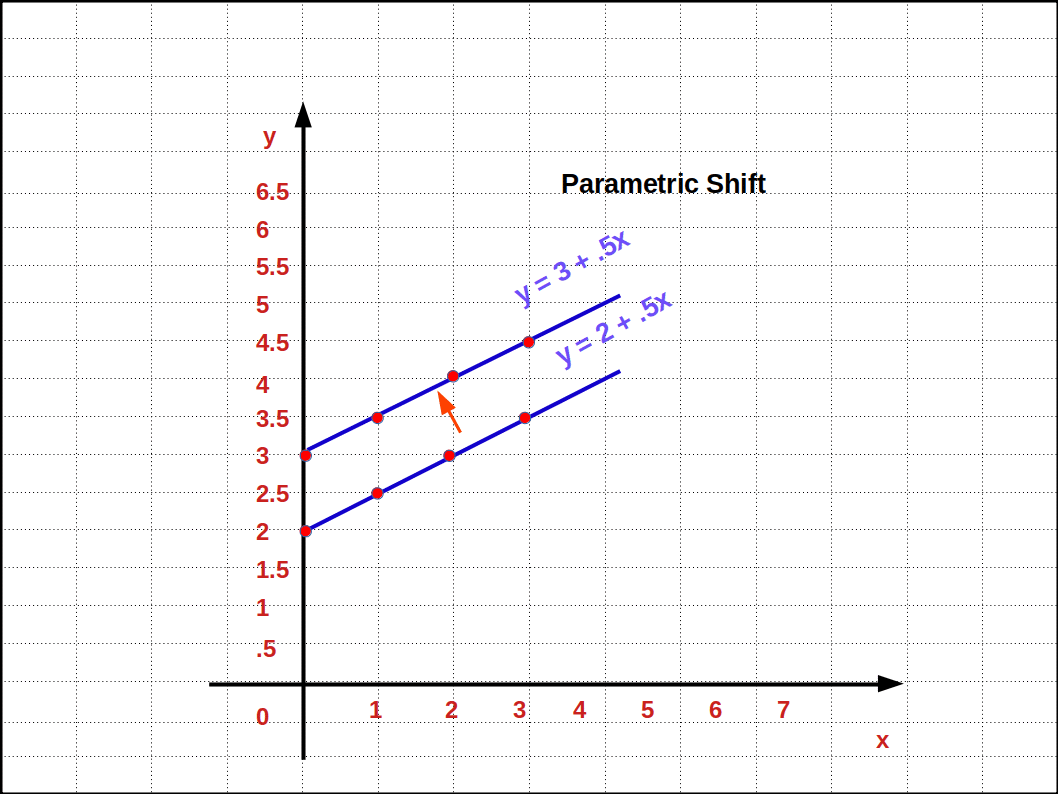Now, suppose that the value of b increases from .5 to 1 keeping value of a as 2. Then, two straight lines having different slopes equal to these values are formed. Thus the straight line swings up as seen in below Graph.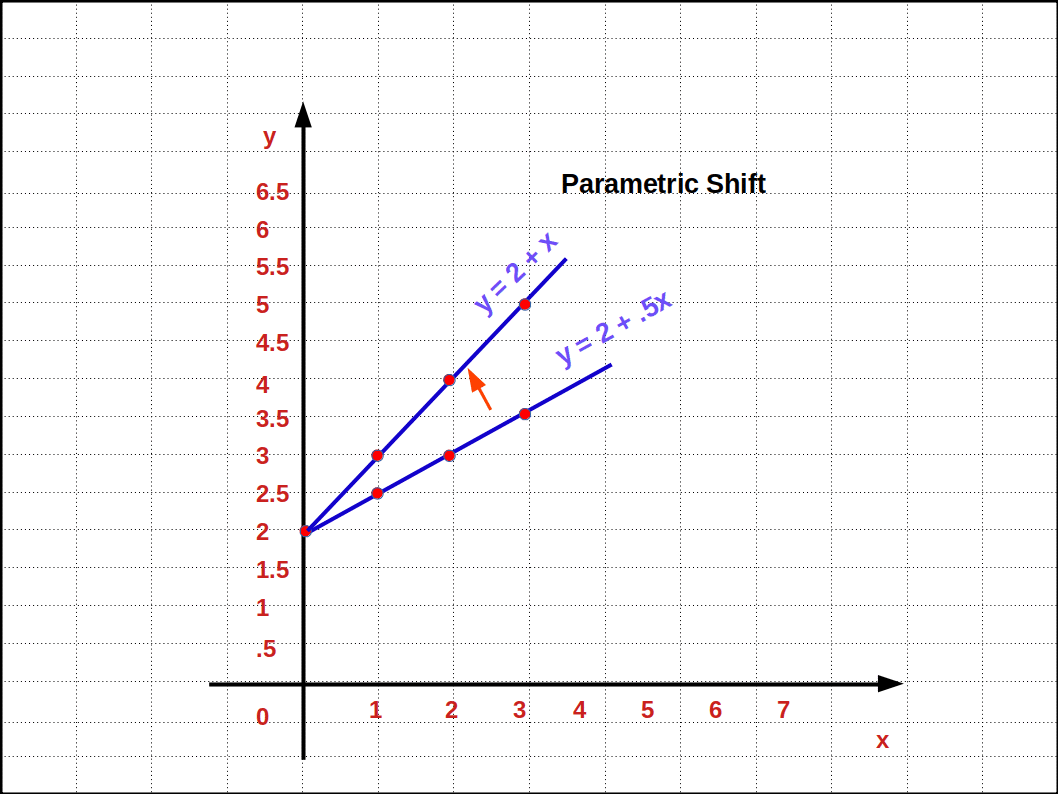## Ex Ante and Ex Post

Terms like consumption, investment, or total output of final goods and services in an economy (GDP) are expressed in two ways. They are ex ante and ex post values. The planned or intended value of a variable is called ex ante measure or value. The actual or realised value of a variable is called ex post measure or value.

For example, a consumer planned to consume goods worth ₹ 10,000 during the year. But actually he consumed goods worth ₹ 8,000 only. In this example, ex ante consumption is ₹ 10,000 and ex post consumption is ₹ 8,000.

In a theoretical model of the economy the ex ante values of the variables are more important. If anyone wants to predict the equilibrium value of GDP, it is very important to know the ex ante values. Therefore, we shall study the determinants of ex ante consumption, investment, saving, aggregate output, etc.

## Ex Ante Consumption

People use a major portion of the income for consumption and the rest as saving. Therefore, income is the sum of consumption and savings. Symbolically,

$$\mathbf{Y \,= \,C \,+ \,S}$$

where, Y = ex ante income,

C = ex ante consumption and

S = ex ante saving.

What does planned consumption depend on? It depends on the size of income of the household and marginal propensity to consume (MPC). Income is the important factor that determines consumption. Therefore, consumption can be taken as a function of income. Mathematically,

$$\mathbf{C \,= \,f(Y)}$$

The relation between consumption and income is called consumption function. The relationship between income and consumption is positive. If income increases consumption also increases and if income decreases, consumption also decreases, but increase in consumption is not proportionate increase in income. The proportion or percentage of income spent on consumption is called MPC. MPC can be defined as the ratio of change in consumption to change in income.

$$\mathbf{MPC\,=\frac{ΔC}{ΔY}}$$

where,

ΔC = change in consumption and

ΔY = change in income.

The proportion of additional total income used for saving is called Marginal Propensity to Save (MPS). MPS can be defined as the ratio of change in, saving to change in income, Symbolically,

$$\mathbf{MPS\,=\frac{ΔS}{ΔY}}$$

where, ΔS = change in saving and

ΔY = change in income.

MPC + MPS = 1

MPC = 1 – MPS

MPS = 1 – MPC

Suppose, when the income of a household increases from ₹ 100 to ₹ 120, consumption increases from ₹ 80 to ₹ 95.

$${MPC\,is\,\frac{ΔC}{ΔY}}$$ = $${\frac{15}{20}}$$ = 0.75

$${MPS\,is\,\frac{ΔS}{ΔY}}$$ = $${\frac{5}{20}}$$ = 0.25

MPS can also be calculated in another way.

MPS = 1 – MPC = 1 – 0.75 =0.25.

• An individual’s income increases from ₹ 1,000 to ₹ 1,500. Then his consumption increases from ₹ 800 to ₹ 1,150.

(1) Find MPC

(2) What is the change in saving ?

(3) Find MPS.

• (1) ΔY = 1,500 – 1,000 = ₹ 500

ΔC = 1,150 – 800 = ₹ 350

$${MPC\,is\,\frac{ΔC}{ΔY}}$$ = $${\frac{350}{500}}$$ = 0.7

(2) ΔS = ΔY – ΔC = 500 – 350 = ₹ 150

ΔC = 1,150 – 800 = ₹ 350

$${MPS\,is\,\frac{ΔS}{ΔY}}$$ = $${\frac{150}{500}}$$ = 0.3

• MPC of a country is 0.75 and MPS = 0.25. Interpret.
• 75% of the increase in income is used for consumption and 25% is used for saving.

When an economy develops, MPC usually falls and MPS rises, Developed countries increase their savings in proportion to their increased income. Poor countries won’t be able to do so.

We saw that increase in consumption is not proportionate increase in income. Consumption increases MPC times of the increased income. Let c be the MPC of an economy. Its value lies between O and one (0 < c < 1). Suppose the total income of the economy increases from 0 to Y. Then the total consumption is

$$\mathbf{C \,= \,c(Y\,-\,0)\,=\,cY}$$

In this equation if income is zero, consumption will also be zero. But this is not practically correct, because, if income is zero, people will have to starve. This is not true. If income is 0 people will buy necessaries with past savings or they will borrow. So we have to add minimum consumption to the above equation. Then the equation becomes

$$\mathbf{C \,= \,\overline{C}\,+\,cY} —(1)$$

In this equation $$\overline{C}$$ > 0. This is the minimum consumption. This is known as autonomous consumption expenditure. Minimum consumption does not depend on the level of income.

If Y= 0, C will be equal to $$\overline{C}$$.

If the income is above 0, the economy uses c proportion of this extra income for consumption over the minimum consumption.

Suppose the consumption equation is C = ₹ 20 + 0.8Y. In this equation income is 0, 20, 40, 60, 80, 100, 120, 140, 160, 180 and 200. Consumption expenditure and saving are given in Table 10.2.

Table 10.2
Income Consumption

(C = 20 + 0.8Y)

Saving

(S = Y – C)

0 20 – 20
20 36 – 16
40 52 – 12
60 68 – 8
80 84 – 4
100 100 0
120 116 4
140 132 8
160 148 12
180 164 16
200 180 20

This consumption schedule clearly shows positive relationship between ex ante consumption expenditure and ex ante income. Increased consumption expenditure is less than increased income.

Consumption and Saving at different income levels are drawn in below Graph as given in Table 10.2.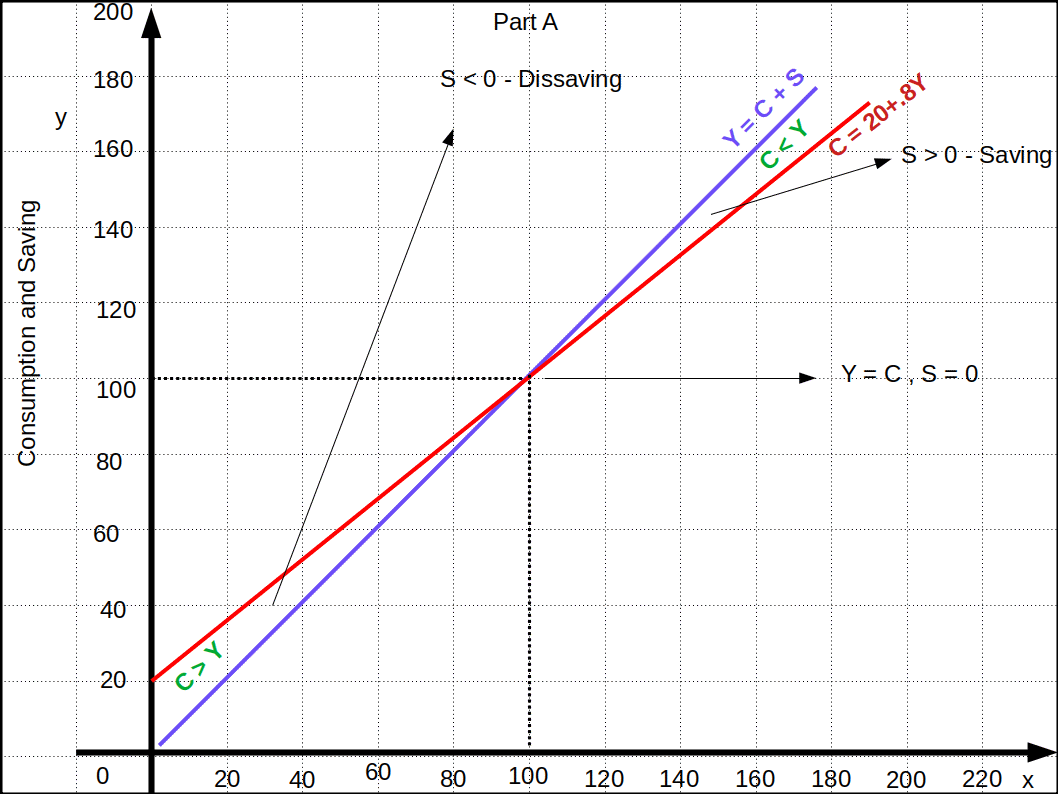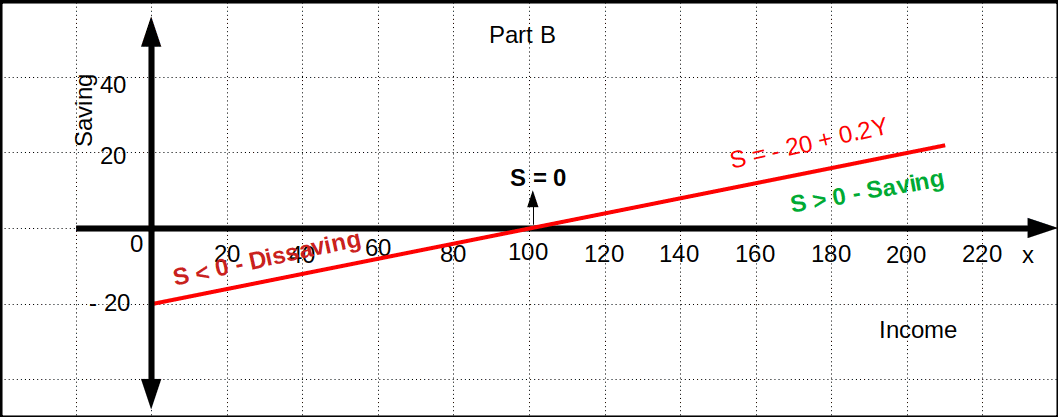There are two parts, A and B. Part A shows the consumption function and Part B Saving function. The line at 45° Y = C + S shows equality line. At any Point on this line, income is the sum of consumption expenditure and Saving. The consumption function line, C = 20 +.8Y shows that when income increases consumption also increases. The vertical intercept of consumption line is 20 and slope is .8. The slope of the 45° line is 1. So, it is clear that increase in consumption is not proportionate to increase in income, and consumption increases at a lower rate than income. At point b income and consumption are equal. The line S = – 20 + .2Y shows the saving function. Till income reaches 100 consumption is more than income and saving is negative. Saving begins from – 20 and increases as income increases.

## Ex Ante and Ex Post

Ex Ante investment is the second component of aggregate demand. It can be defined.as addition to the stock of physical capital and changes in inventory of all the producers in the economy.

Investment demand mainly depends on rate of interest and Marginal Efficiency of Capital (MEC). Investment demand is a function of interest rate and MEC. But, for simplicity, we assume that firms plan to invest the same amount every year. We assume that the investment demand J as autonomous investment in the economy.

So,

$$\mathbf{I = \overline{I}} — (2)$$

MEC is the expected annual returns of an additional unit of investment.

## Ex Ante Aggregate Demand (AD)

Ex ante aggregate demand for final goods is the total demand for final goods and services in an economy. This is the aggregate final expenditure of the economy. Ex ante aggregate demand for final goods in a closed €conomy without Government is the sum of ex ante consumption expenditure and ex ante investment expenditure.

In short,

$$AD\,=\,C\,+\,I$$

The value of C and I in the equations (1) and (2) can be substituted here to get the ex ante aggregate demand for final goods.

$$\mathbf{AD\,=\overline{C}+cY+\overline{I}\,=\,\overline{C}+\overline{I}+cY}$$ $$\mathbf{AD\,=\,\overline{A}+cY}—(3)$$

Where, $$\overline{A}\,=\,\overline{C}\,+\,\overline{I}$$. This is the total autonomous expenditure.

Equation (3) is the ex ante aggregate demand equation of a two sector economy, consisting of household sector and the firms. If this equation is exhibited in a diagram it will be a straight line that goes upwards with vertical intercept $$\overline{A}$$ and positive slope c. This is the AD line/curve and is shown in below Diagram.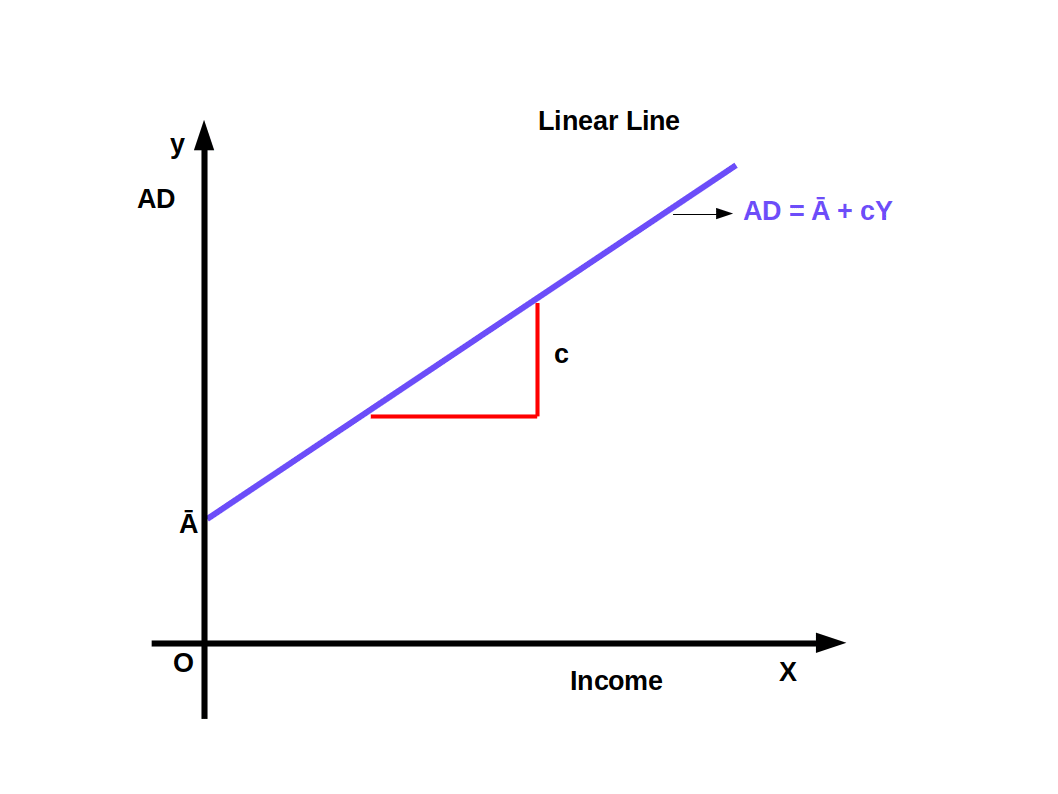Suppose the consumption function of an economy is C = ₹ 20 + .8Y. Its ex ante investment is I = Ī = ₹ 20. Then AD = C +I. AD = 20 + .8Y + 20 = 40 + .8Y, AD = ₹ 40 + .8Y. This is the ex ante aggregate demand equation. Using this equation we can calculate the ex ante aggregate demand on the basis of ex ante income at different levels. For example, the AD is given in the following table for income ₹ 0, ₹ 100, ₹ 200, ₹ 300.

Table 10.3
Income

Y

Aggregate demand

Method of finding Y
0 40 AD = 40+0.8×0 = 40+0 = 40
100 120 AD = 40+0.8×100 = 40+80 = 120
200 200 AD = 40+0.8×200 = 40+160 = 200
300 280 AD = 40+0.8×300 = 40+240 = 280

• Consumption function, C = ₹ 50 + -75Y and investment I = Ī = ₹ 40. Frame the aggregate demand equation.
• AD = C + I

= 50 + .75Y + 40

AD = ₹ 90 + .75Y

## Ex Ante Aggregate Supply (AS)

Ex Ante Aggregate Supply is the total goods and services planned to be supplied in the economy. This is the aggregate national product/national income. National income, planned aggregate output and planned aggregate income are different terms used to convey the same meaning. i.e., AS = Y. AS = Y will be a Straight with 45° positive slope as seen in below Diagram.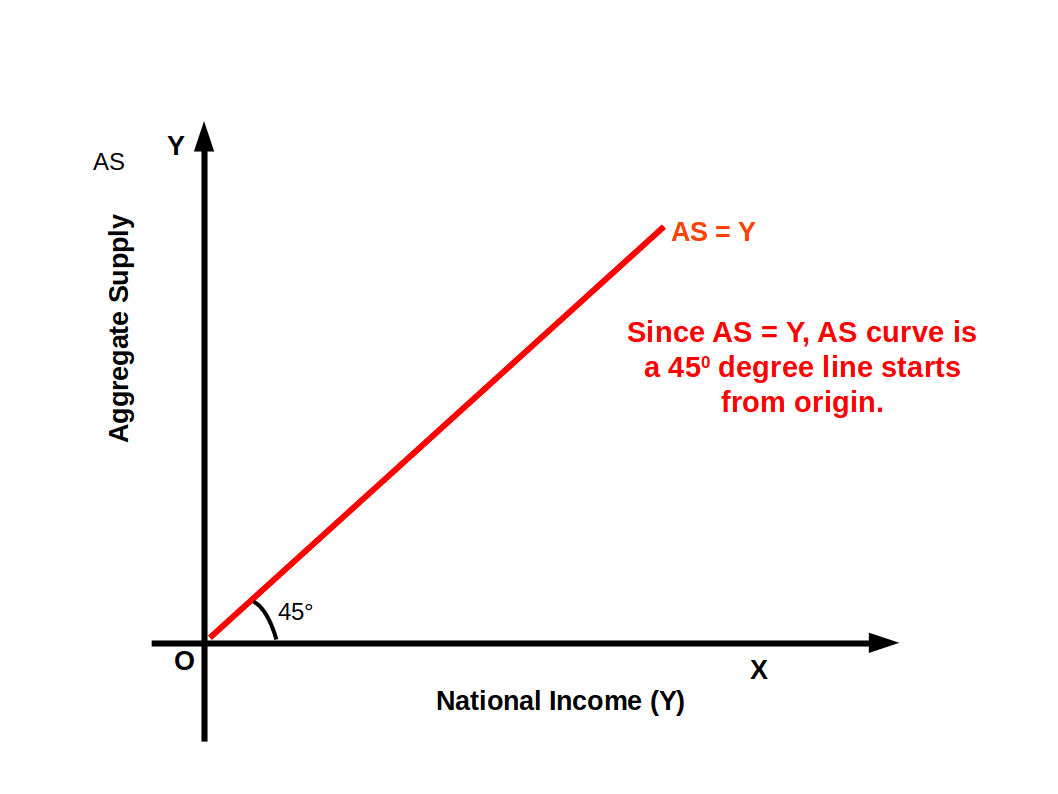## Short run Fixed Price Analysis of the Product Market

In an economy, when price and interest rate remain fixed, AD – AS curves intersect and determine equilibrium income. Now we shall discuss how it is done.

Producers supply all goods demanded by the consumers in order to keep the price steady in the higher than the quantity of demand or less, it creates either excess supply or excess demand. As a result of this price changes. To solve this problem we suppose that the elasticity of supply is infinite. That is, at fixed price, supply curve will be horizontal. But AD curve will be sloping down compared to price level.

In the short run, when price is fixed the only factor that determines equilibrium income is AD curve. That is, since short run supply curve is perfectly elastic, AD curve determines the equilibrium income. This principle is known as Effective Demand Principle. Equilibrium Income is the income at which AS and AD are equal.

In the below Diagram AS is aggregate supply curve and AD is aggregate demand curve. AD curve intersects AS curve at point E. E is the point of effective demand, where AS and AD are equal. Therefore, equilibrium income or output is Y*.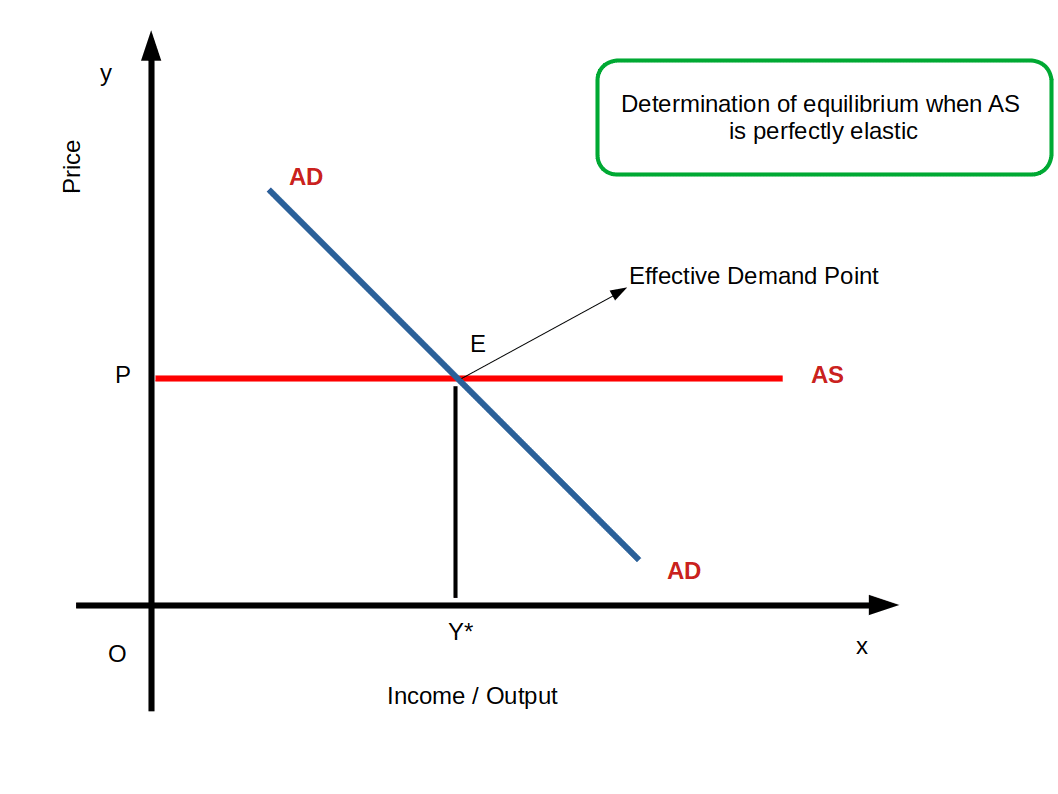## A Point in the Aggregate Demand Curve

At a fixed price the value of AD for final goods is the sum total of ex ante consumption expenditure and ex ante investment expenditure. Under the effective demand principle, the equilibrium level of output of final goods equals ex ante aggregate demand.

Algebraically,

Y = Ᾱ + c.Y

Where Y = Aggregate output

Ᾱ + c.Y = Aggregate demand

Let us consider an example to derive the value of AD and hence equilibrium output at a fixed price. Take value of autonomous expenditure as $$\overline{C}$$ = 40, I = Ī = 10, the value of mpc (c) = 0.8 and Y = 200.

To find equilibrium of the economy

Y = $$\overline{C}$$ + Ī + c.Y

200 = 40 + 10 + 0.8 × 200

200 = 50 + 0.8 × 200

200 = 50 + 160

200 = 210

i.e., 200 ≠ 210

Here the economy is not in equilibrium because aggregate output (200) is not equal to aggregate demand (210). i.e., aggregate demand greater than aggregate output denotes the situation of excess demand. Suppose the income increases to 250, the Ᾱ, mpc are constant

Y = Ᾱ +cY

250 = 50 + 0.8 × 250

250 = 50 + 200

250 = 250

Hence we have ultimately hit the correct value of Y where AD equals aggregate output. So the equilibrium level of output of the economy at the fixed price is 250 i.e., Y = 250.

## Determination of Equilibrium Income and Output in a Closed Economy

In a closed economy equilibrium income and equilibrium output are determined by Effective Demand. Effective demand is a situation in which ex Ante aggregate supply and ex ante aggregate demand become equal. Therefore, the product market reaches equilibrium when AS and AD are equal in an economy.Mathematically,

$$\mathbf{Equilibrium:\, AS = AD}\,or\,\mathbf{Y = AD}$$

$$\mathbf{Y\,=\,Ᾱ\,+\,cY}- – -(4)$$

In an economy, since the ex ante aggregate demand for final goods is the sum of ex ante aggregate consumption demand and ex ante investment demand,

Y = C + I (Since AD = C + I)

Y = $$\overline{C}$$ + cY + Ī and

Solve for Y

Y – cY = $$\overline{C}$$ + Ī

Y(1-c) = $$\overline{C}$$ + Ī

$$\mathbf{∴\, Y\,=\,\frac{\overline{C}\,+\,\overline{I}}{1\,-\,c}}$$

If Y* is the equilibrium income.

$$\mathbf{∴\, Y\,=\,\frac{\overline{C}\,+\,\overline{I}}{1\,-\,c}} \,=\,\mathbf{\frac{\overline{A}}{1\,-\,c}}$$

where $$\overline{A}\,=\, \overline{C}\,+\,\overline{I}$$

## Determination of Equilibrium Income and Output: A Diagram

We shall explain the determination of equilibrium income/output in an economy with the help of a diagram.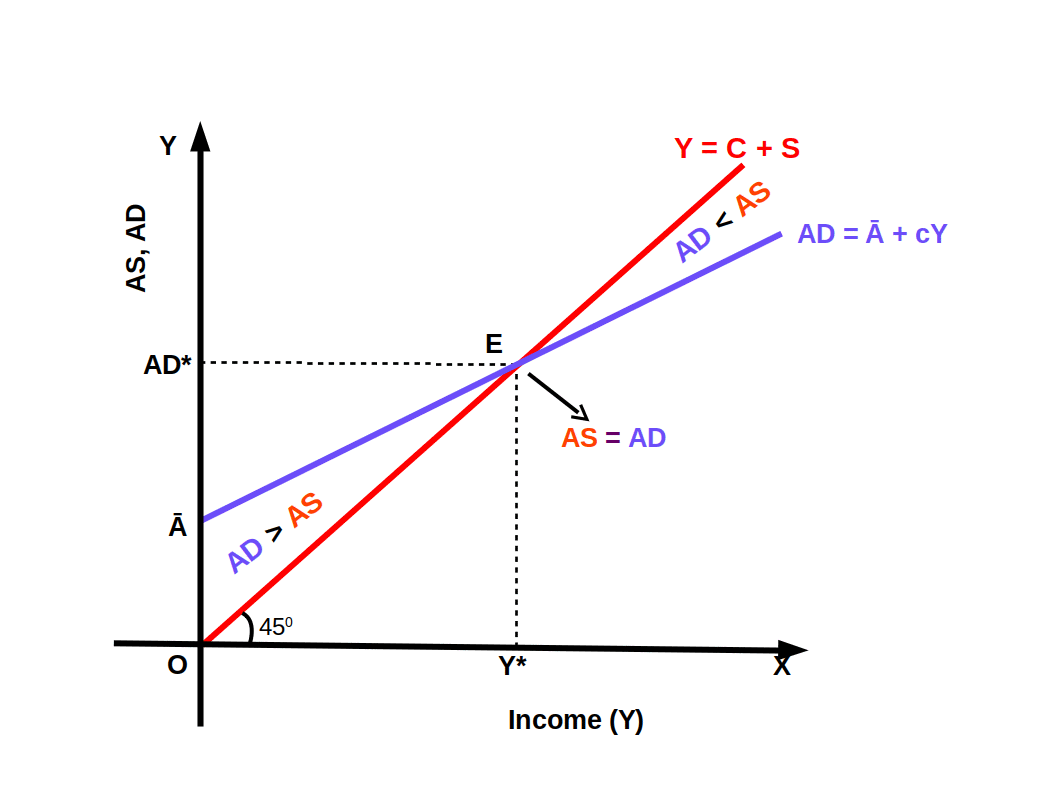In the above Diagram, ex ante income is marked on the x-axis, ex ante aggregate demand and ex ante aggregate supply are marked on y axis. 45° line Y = C + S is the aggregate supply line. At any point on this line, AS and AD would be equal. AD = Ᾱ + cY is the aggregate demand line. The vertical intercept of this line is Ᾱ and slope is c.

AD is more than AS before income level Y*, This shows excess aggregate demand. After income level Y*, AD is less than AS. This indicates deficiency in AD. But when income is Y*, AS and AD are equal. Point E is the point of effective demand. At this point there is neither excess demand nor deficient demand, Then equilibrium income is Y* and equilibrium demand is AD*.

Suppose C = ₹ 20 + .8Y and I = Ī = ₹ 20. Equilibrium condition is

$${ Y\,=\,\frac{\overline{C}\,+\,\overline{I}}{1\,-\,c}} {\,=\,\frac{20\,+\,20}{1\,-\,.8}}$$

$$Y\,=\,\frac{{40}}{1\,-\,.8} \,=\,\frac{{40}}{.2}$$

= ₹ 200

• In an economy, Autonomous consumption expenditure is ₹ 200 crore. Autonomous investment is ₹ 100 crore. Marginal propensity to consume is 0.7. Calculate equilibrium income.
• $${ Y*\,=\,\frac{\overline{C}\,+\,\overline{I}}{1\,-\,c}} {\,=\,\frac{200\,+\,10}{1\,-\,.7}}$$

$$=\,\frac{{300}}{1\,-\,.7} \,=\,\frac{{300}}{.3}$$

= ₹ 1000 crore

## Effect of Autonomous Change on Equilibrium Demand in the Product Market

When change occurs in the autonomous component, what happens to equilibrium demand and equilibrium income? Let us discuss.

Suppose aggregate demand line AD before change in the autonomous component is AD1 = Ᾱ1 + cY. This is given in below Diagram. Line AD, cuts AS curve at point E1. Then Y*1 is the equilibrium output. AD1* is the equilibrium demand. Now there is an increase in the autonomous investment by Δl. Then aggregate demand equation is AD1 = Ᾱ1 + cY + Δl. This new demand equation can be written as AD2 = Ᾱ2 + cY.Due to increase in autonomous investment, a new aggregate demand curve/line AD2 shifts upward parallel to AD1. Vertical intercept increases Ᾱ1 from to Ᾱ2 without any change in the slope of demand curve. Line AD2 cuts line AS at the new point E2 and Y2 becomes the new equilibrium income. As a result of increase in autonomous investment by ΔI = E1F, equilibrium demand increases from AD1* to AD2* and equilibrium income/output increases from Y1* to Y2* (ΔY = E1G).

We can summarise the change in equilibrium demand and equilibrium income due to change in autonomous component in the following manner.

###If there is an increase in autonomous expenditure, aggregate demand curve will shift parallel upward and equilibrium demand and income will increase.

If there is a decrease in autonomous expenditure, aggregate demand curve will shift parallel downwards and as a result equilibrium demand and output will decrease.

Suppose the consumption function of an economy is C = ₹ 20 + .8Y and investment expenditure is I = Ī = ₹ 20.

AD1 = 20 + .8Y + 20; AD1 = 40 + .8Y

Y = 40 + .8Y

Y – .8Y = 40

.2Y = 40

$$Y\,=\,\frac{40}{.2}$$

Y1* = ₹ 200

Now suppose that autonomous investment increases by ₹ 10. Then aggregate demand line is AD2 = AD1 + 10 = 40 + .8Y + 10; AD2 = 50 + .8Y. In equilibrium, Y = AD2 = 50 + .8Y; Y – .8Y = 50, .2Y = 50; $$Y\,=\,\frac{40}{.2}$$ Equilibrium output, Y2* = ₹ 250. In this example, when autonomous investment increased by ₹ 10 equilibrium output increased from ₹ 200 to ₹ 250.

## Effect of MPC on Equilibrium Demand

The autonomous factor and MPC are the two components of aggregate demand line AD = Ᾱ + cy; AD line will swing up or down with change in the second factor c. If MPC increases the slope of line AD will increase and swing up. This is shown in below Diagram.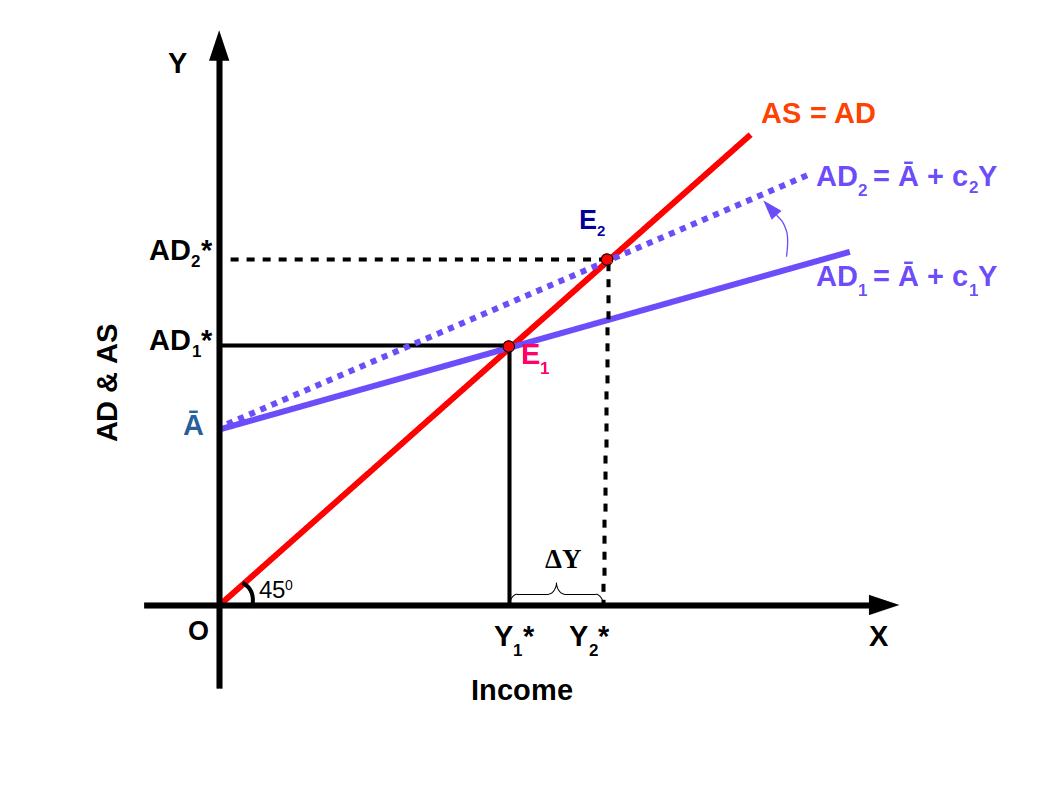AD1 = Ᾱ + c1Y, is the AD line before the increase in MPC. AD2 = Ᾱ + c2Y is the AD line after the increase in MPC. Since c2 is greater than c1 aggregate demand line swings up as AD2 as seen in the diagram. As a result of increase in the value of MPC from c1 to c2 equilibrium point moves from E1 to E2. Equilibrium demand increases from AD1* to AD2*. Then the new equilibrium output increases from Y1* to Y2*.

Suppose aggregate demand line is AD1 = 50 + -5Y. Then equilibrium output Y1* is 100.

When MPC increases from .5 to .8 aggregate demand line AD2 = 50 + .8Y and the equilibrium output at this point is 250. In this example, autonomous expenditure is the same, 50. When MPC increases from .5 to .8 equilibrium output increases from 100 to 250 and is shown in below Graph.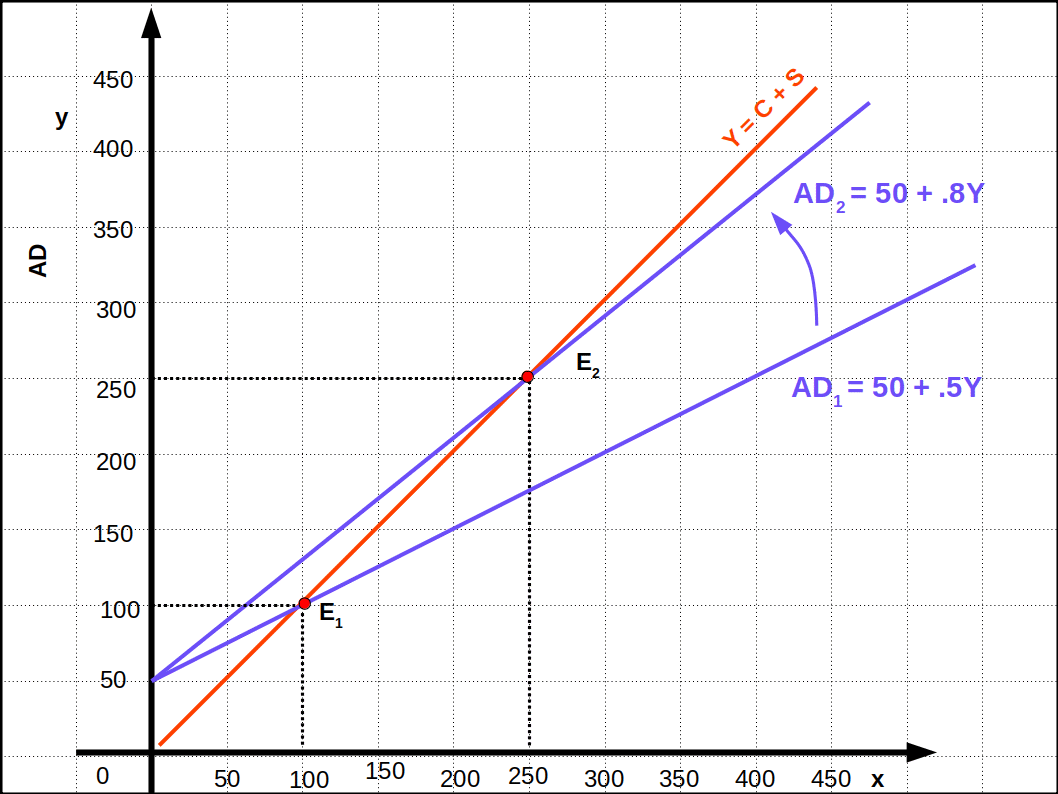Change in MPC can be summarized as follows:

###If MPC increases, AD line will swing upwards and equilibrium aggregate demand and equilibrium output will increase.

If MPC falls, AD line will swing downwards, and both equilibrium demand and output will decrease.

## Output Multiplier

Output multiplier is the ratio of change in output to the change in autonomous expenditure. Mathematically,

$$\mathbf{K\,=\,\frac{ΔY}{ΔᾹ}}—(5)$$

where, K = Output multiplier, ΔY = change in output/income, ΔᾹ = Change in autonomous expenditure.

Output multiplier is also known as Income Multiplier and Autonomous Expenditure Multiplier.

$$\mathbf{ΔY\,=\,KΔᾹ}$$ We know that in a closed economy, equilibrium output Y* is $$\frac{Ᾱ}{1-c}$$.Y* is the product of $$\frac{1}{1-c}$$ and Ᾱ. This implies that equilibrium output changes by $$\frac{1}{1-c}$$ is called closed economy autonomous expenditure multiplier. Then equation (5) can be written as given below:

$$\mathbf{K\,=\,\frac{ΔY}{ΔᾹ} \,=\, \frac{1}{1-c}}$$

$$or$$

$$\mathbf{ΔY\,=\,\frac{ΔᾹ}{1-c}}$$

$$\mathbf{K\,=\,\frac{ΔY}{ΔᾹ} \,=\, \frac{1}{1-MPC} \,=\,\frac{1}{MPS}}$$

Suppose in an economy, autonomous expenditure increases from ₹ 40 to ₹ 50, equilibrium output increases from ₹ 200 to ₹ 250. Then output multiplier is $$\frac{ΔY}{ΔᾹ} = \frac{50}{10}$$ = 5. That is, when autonomous expenditure increased by ₹ 10, equilibrium output increased by ₹ 50. This is 5 times increase of autonomous expenditure (50 =5 × 10).

Suppose MPC of an economy is .8. Then multiplier is $$\frac{ΔY}{ΔᾹ}$$ = $$\frac{1}{1-c}$$ = $$\frac{1}{1- .8}$$ = $$\frac{1}{.2}$$ = 5.

We can calculate the ex ante national income, consumption and saving of an economy if we know the autonomous expenditure $$\overline{C}$$, $$\overline{I}$$ and MPC or MPS.

Suppose in an economy, $$\overline{C}$$ = ₹ 20, $$\overline{I}$$ = ₹ 30, MPC= .8.

Then, $$K\,=\, \frac{1}{1-c}$$ = $$\frac{1}{1-.8}$$ = $$\frac{1}{.2}$$ = $$\frac{10}{2}$$ = 5

Equilibrium output Y is, $$Y\,=\,\frac{1}{1-c}$$ × Ᾱ, or Y = K × Ᾱ

where $$\overline{Ᾱ}$$ = $$\overline{C}$$ + $$\overline{I}$$ = 20 + 30 = 50

Then, Y = 5 × 50 = 250

That is, Y = ₹ 250

From this we can find the consumption expenditure.

We know that C = $$\overline{C}$$ + cY

∴ C = 20 + .8Y = 20 + .8 × 250

= 20 + 200 = ₹ 220

## The Process of Multiplier Mechanism

Suppose the consumption function of an economy is C = ₹ 20 + .8Y and investment expenditure is I = Ī = ₹ 20.

AD1 = 20 + .8Y + 20; AD1 = 40 + .8Y

Y = 40 + .8Y

Y – .8Y = 40

.2Y = 40

$$Y\,=\,\frac{40}{.2}$$

Y1* = ₹ 200

Now suppose that autonomous investment increases by ₹ 10. Then aggregate demand line is AD2 = AD1 + 10 = 40 + .8Y + 10; AD2 = 50 + .8Y. In equilibrium, Y = AD2 = 50 + .8Y; Y – .8Y = 50, .2Y = 50; $$Y\,=\,\frac{40}{.2}$$ Equilibrium output, Y2* = ₹ 250. In this example, when autonomous investment increased by ₹ 10 equilibrium output increased from ₹ 200 to ₹ 250.

In the above problem, income increased by ₹ 50 (ΔY = 50) as a result of an increase in autonomous expenditure by ₹ 10 (ΔI = 10). This is due to multiplier effect. Now we shall discuss how this happens.

The process of multiplier mechanism is very interesting. An increase in autonomous expenditure causes an increase in the aggregate demand. This leads to excess demand. In our example, since the excess autonomous expenditure is ₹ 10(Y= AD = 200), aggregate demand increases from ₹ 200 to ₹ 210. That is, excess demand is ₹ 10. The producers reduce their inventory and deal with the excess demand.

In the next production cycle, the producers plan to increase their output by ₹ 10. When: they produce goods worth ₹ 10, they distribute it to the owners of production factors in the form of factor payment. Then, in the first round of multiplier process, income of factors of production increases by ₹ 10.

The propensity of the people to consume is 0.8. That means 80% of the income received is used for consumption. People use .8 times × ₹ 10 (.8 × 10) for consumption in the second round. So, AD increases to .8 × 10. Again excess demand equal to .8 × 10 comes up.

Thus, in the next production cycle, the producers again increase the ex ante output by .8 × 10. By this the equilibrium condition is restored. When this income out of production is distributed among the factors of Production, the income of the economy goes up by .8 × 10.

This process goes on for several rounds and size of the increment will be indistinguishable from zero and subsequent round effects will not practically contribute anything to the total volume of output. The process of diminishing AD and reaching nearer to 0 is called convergent process. Different rounds of multiplier process are given in Table 10.4.

Table 10.4
Rounds Consumption AD AS = Y
Round 1 0 10 10
Round 2 .8(10) .8(10) .8(10)
Round 3 .82(10) .82(10) .82(10)
Round 4 .83(10) .83(10) .83(10)
.

.

.

.

.

.

.

.

Total 50 50

To find the sum total of increase in final output it is enough to add the infinite geometric series given in the last column of Table 10.4.

ΔY = 10 + (.8) 10 + (.8)2 10 + (.8)3 10 + … ∞

= 10 [1 + (.8) + (.8)2 + (.8)3 + …. ∞]

= 10 $$\Bigl( \frac{1}{1-.8}\Bigr)$$ = 10 $$\Bigl(\frac{1}{.2}\Bigr)$$ = 10 × 5 = 50

Initially, with ₹ 10 increase in autonomous expenditure, there is an increase of ₹ 50 in output due to multiplier process. This is 5 times the autonomous expenditure.